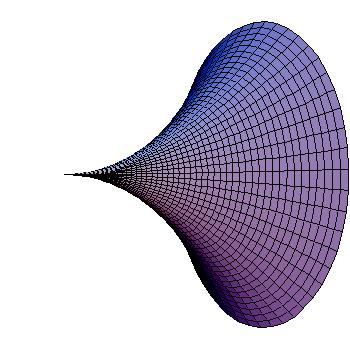# A calculus problem by Kunal Gupta

Calculus Level 4Consider the curve $y={ e }^{ -x }$ in the first quadrant. Now it's rotated about the $x\text{-axis}$ to obtain a solid of revolution. What is its surface area to 4 decimal places?

×